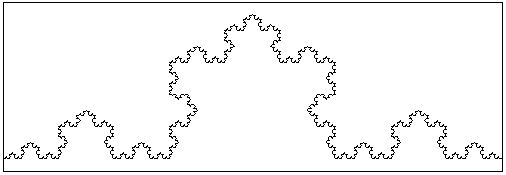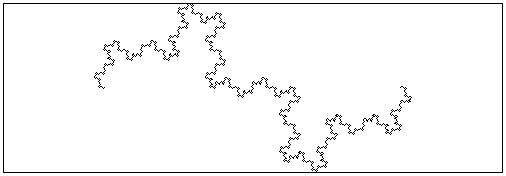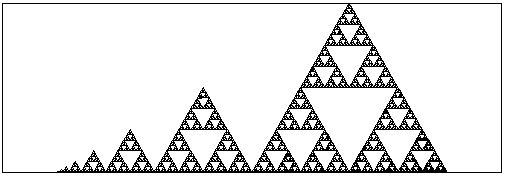# Fractal Curves and Dimension

Fractals burst into the open in early 1970s. Their breathtaking beauty captivated many a layman and a professional alike. Striking fractal images can often be obtained with very elementary means. However, the definition of fractals is far from being trivial and depends on a formal definition of dimension.

It takes a few chapters of an Advanced Analysis book to rigorously define a notion of dimension. The important thing is that the notion is not unique and even more importantly, for a given set, various definitions may lead to different numerical results. When the results differ the set is called fractal. Or in the words of Benoit Mandelbrot [Ref 2], the father of fractals:

A fractal is by definition a set for which the Hausdorff-Besicovitch dimension strictly exceeds the topological dimension.

The topological dimension of a smooth curve is, as one would expect, one and that of a sphere is two which may seem very intuitive. However, the formal definition was only given in 1913 by the Dutch mathematician L. Brouwer (1881-1966). A (solid) cube has the topological dimension of three because in any decomposition of the cube into smaller bricks there always are points that belong to at least four (3+1) bricks.

The Brouwer dimension is obviously an integer. The Hausdorff-Besicovitch dimension, on the other hand, may be a fraction. Formal definition of this quantity requires a good deal of the Measure Theory. But fortunately for a class of sets Hausdorff-Besicovitch dimension can be easily evaluated. This sets are known as the self-similar fractals and, because of that ease, the property of self-similarity is often considered to be germane to fractals in general. The applet below illustrates the idea of self-similarity.

Initially there is an equilateral triangle in the middle of a straight line segment. This combination of four segments form a pattern which serves as a Description of an algorithm to be used later on. The secret meaning of the pattern is this

Start with a straight line segment. Proceed in stages. On every stage traverse the curve obtained previously. Every time you encounter a straight line segment, replace it with the four-segment pattern as was first done with the original line segment.

As with the Hilbert curve, it's possible to formally prove that thus obtained curves converge to a limit curve. This limiting curve is self-similar because it consists of four smaller copies of itself which, in turn, also and naturally consist of four smaller copies of themselves and so on. To see how the algorithm works click on the applet and watch. Click for an additional demonstartion. What you see is the formation of the curve known as the Koch's snowflake (see also Koch's Snowflake for another implementation).

### If you are reading this, your browser is not set to run Java applets. Try IE11 or Safari and declare the site https:///www.cut-the-knot.org as trusted in the Java setup.What if applet does not run?

To understand the notion of the similarity dimension, first observe that, if the initial line segment was 1 unit in length, then the second stage curve that consists of four segments each one third of the initial line, is 4/3 units in length. On the third stage the length becomes (4/3)2 and on the fourth it's (4/3)3 and so on. Since 4/3>1 the farther you go the longer the curve results. Finally, the limiting curve will have infinite length. Which is somewhat surprising because of it's being generated by such a simple procedure in a very limited space from the curves of finite length.

The similarity dimension of the snowflake curve is finite. This is arrived at in the following manner. If it were a straight line we could split it into two smaller segments each half the length of the "parent" line. The length of the line would be the sum of the two smaller segments. If we were talking about areas, then taking a square and splitting it into 4 smaller squares with areas 1/4 of the "parent" square. We would observe that the four smaller areas sum up to the original size. Notice that when the side of a square is halved, its area decreases by the factor of 4 which is (1/2)2. For a cube, acting similarly, decreasing its side by a factor of 2 results in smaller cubes each with the volume 1/8 = (1/2)3 of the "parent" cube. We can detect a commonality in these three examples. Given a shape of size S. It's split into N similar smaller shapes each with the size S/N so that N·(S/N) = S. In each of the three cases we used a different function S. If a is a linear dimension of the shape we have S(a) = a for a line segment and S(a) = a2 and S(a) = a3 for the square and cube, respectively. Thus, N·(S/N) = S can be rewritten as N·(a/M)D=aD where a is the "linear size" of the shape, M is the number of linear parts, and N is the total number of the resulting smaller shapes. This gives N·M-D = 1 or N = MD. In all three cases we took M = 2 and D was successively 1, 2, and 3. We see that D=log(N)/log(M) is what we would call the dimension in all three cases.

This quantity D is known as the similarity dimension. It applies to shapes that are composed of a few copies of themselves whose "linear" size is smaller than that of the "parent" shape by a factor of M. Returning to the snowflake, we have N = 4 and M = 3. In this case D = log(4)/log(3) is somewhere between 1 and 2.

The Koch's snowflake has no self-intersection and is obtained from a line segment as an image of a continuous function. By one of Brouwer's theorems this function preserves the topological dimension of the segment (which is, of course 1). Finally, the curve has topological dimension 1 whereas its Hausdorff-Besicovitch dimension is log(4)/log(3).

Note that for the Hilbert curve that (disregarding small links) consists of 4 (= N) copies of itself each twice (M = 2) as short as the whole, we get D = log(4)/log(2) = 2. Which is not surprising as the curve covers the whole of the square whose dimension is 2. From the above mentioned Brouwer's theorem it follows that the limiting curve must have self-intersections. For otherwise it would be a topological (in particular, dimension preserving) transformation. But a line is of (topological) dimension 1 whereas a square has dimension 2.Here is another example. In this case, N=8 and M=4. Therefore, the similarity dimension of the limit curve is D=log(8)/log(4)=3/2. A rare case of rational dimension.

### If you are reading this, your browser is not set to run Java applets. Try IE11 or Safari and declare the site https:///www.cut-the-knot.org as trusted in the Java setup.What if applet does not run?Oops. Talking about rare cases. For the fractal below N=4 and M=4/21/2=23/2. Therefore, D=log(4)/log(23/2)=2·2/3=4/3. (The angles of interest to you are 45o and 90o)

### If you are reading this, your browser is not set to run Java applets. Try IE11 or Safari and declare the site https:///www.cut-the-knot.org as trusted in the Java setup.What if applet does not run?What if applet does not run?### This applet requires Sun's Java VM 2 which your browser may perceive as a popup. Which it is not. If you want to see the applet work, visit Sun's website at https://www.java.com/en/download/index.jsp, download and install Java VM and enjoy the applet.

 What if applet does not run?-->

This is a family of Sierpinski gaskets. What's the dimension of each?

### If you are reading this, your browser is not set to run Java applets. Try IE11 or Safari and declare the site https:///www.cut-the-knot.org as trusted in the Java setup.What if applet does not run?### Reference

• M. Gardner, Penrose Tiles to Trapdoor Ciphers, W. H. Freeman and Co., NY, 1989.
• B. Mandelbrot, The Fractal Geometry of Nature, W. H. Freeman and Co., NY, 1977.

### On Internet### Remark

It's interesting to observe that if a fractal curve serves as a boundary of a plane region the region itself will not be fractal. It will possess a well defined area as a good plane region would.### Credits

The applet above is a modification of an example written by Jim Graham and distributed along with the Java Toolkit.